# Using a LSTM for Linear System Identification

This example shows how to use Long Short-term Memory (LSTM) neural networks to estimate a linear system and compares this to transfer function estimation. The example investigates the ability of an LTSM to capture the underlying dynamics of the system being modelled. To do this, a LTSM is trained on the input and output data from a linear transfer function. Gaussian white noise is used to construct an input signal, the input is applied to a system to generate a corresponding output signal. The input and corresponding output signals are then used to train the network. Once the LSTM is trained it is tasked with estimating the system's response to a step change. The ability of the LSTM to capture the system dynamics is measured by how accurately the system can estimate the system's response to a step change.

LSTM's are a specific type of network from the general class of Recurrent Neural Networks (RNN) that are capable of learning temporal features and long-term dependencies from sequential and time series data. Because of the exploding gradient phenomenon encountered with RNNs, a specific architecture, the LSTM, has emerged to address this issue. Specifically, LSTM units generalize well for capturing temporal dependencies while not suffering from the exploding gradient phenomenon. For this reason, they have been successfully implemented in a wide range of problems raning from handwriting recognition to protein secondary structure prediction.

The first version of the LSTM unit architecture was introduced in 1997 . Since then, various improvements to the architecture have been made: extending LSTM with forget gates , developing bidirectional LSTMs  and deep LSTM .

### Transfer function

This example uses a fourth order transfer function with mixed fast and slow dynamics. The damping of the system is moderate to allow the system dynamics to damp out over a longer time horizon. This is done particularly to investigate the ability of an LSTM to capture the mixed dynamics without some of the important response dynamics damping out. Below, the transfer function is constructed by specifying the poles and zeros of the system.

```fourthOrderMdl = zpk(-4,[-9+5i;-9-5i;-2+50i;-2-50i],5e5); [stepRespone,stepTime] = step(fourthOrderMdl);```

Plot the transfer functions' step response

```plot(stepTime,stepRespone) grid on axis tight title('Fourth order mixed step response')```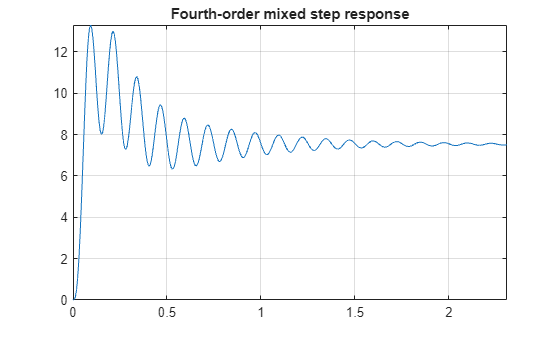The bandwidth of the system (which is measured as the first frequency where the gain drops below 70.79% (~3dB) of its DC value) is

`bodeplot(fourthOrderMdl)`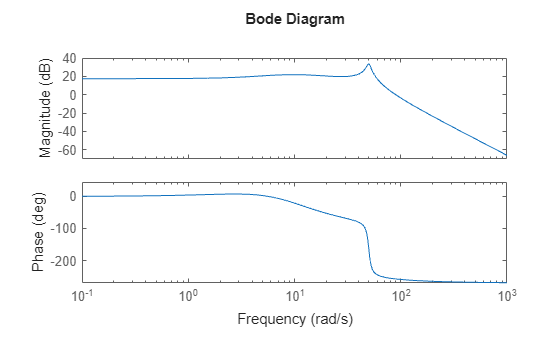`fb = bandwidth(fourthOrderMdl)`
```fb = 62.8858 ```

### Generate training data

In order to explore whether the underlying dynamics are being captured, the LSTM is trained on random gaussian noise. Following this training the network is tasked with predicting the system's response to a step input. The ability of the LSTM to capture the underlying dynamics is therefore measured by its success in predicting the step response. For this process a Gaussian noise signal is generated as input to the transfer functions and the LSTM is trained on the random signal and the corresponding response from the transfer function.

#### Gaussian noise data

A random Gaussian noise signal is generated as a training signal. The signal is sampled 100 times a second and has a duration of 50 seconds

```signalType = 'rgs'; % Gaussian signalLength = 5000; % Number of points in the signal fs = 100; % Sample frequency. signalAmplitude = 1; % Maximum signal amplitude.```

The gaussian noise signal is generated using the in-built `idinput` MATLAB function. The function requires a signal length and a signal type to be specified. Here, `rgs` is selected to generate random Gaussian noise.

Generate and scale the training signal

```urgs = idinput(signalLength,signalType); urgs = (signalAmplitude/max(urgs))*urgs';```

Generate the time signal based on sample rate

`trgs = 0:1/fs:length(urgs)/fs-1/fs;`

The `lsim` function is used to generate the response of the system. The corresponding response from the system is generated and stored in `yrgs`. The simulated output is transposed to correspond to the LSTM input structure which requires row vectors and not column vectors.

```yrgs = lsim(fourthOrderMdl,urgs,trgs); yrgs = yrgs';```

Similarly, create a shorter validation signal to use during network training.

```xval = idinput(100,signalType); yval = lsim(fourthOrderMdl,xval,trgs(1:100));```

### Training network

The network architecture was determined by using a Bayesian optimization routine where the Bayesian optimization cost function uses independent validation data (see the accompanying `bayesianOptimizationForLSTM.mlx` for the details). Although multiple architectures may work, the computationally most efficient one is sought. Furthermore, it was seen that as the complexity of the transfer function increases, when applying LSTM to other linear transfer functions, the architecture of the network does not change significantly. Rather, the number of epochs needed to train the network increases. The number of hidden units required for modeling a system is related to how long the dynamics take to damp out. In this case there are two distinct parts to the response: a high frequency response and a low frequency response. A higher number of hidden units are required to capture the low frequency response. If a lower number of units are selected the high frequency response is still modeled. However the estimation of the low frequency response deteriorates.

#### Setting up network architecture

```% Network architecture numResponses = 1; featureDimension = 1; numHiddenUnits = 100; maxEpochs = 1000; miniBatchSize = 200; Networklayers = [sequenceInputLayer(featureDimension) ... lstmLayer(numHiddenUnits) ... lstmLayer(numHiddenUnits) ... fullyConnectedLayer(numResponses) ... regressionLayer];```

Setting the network initial learning rate is important; using an initial learning rate that is too high results in high gradients which leads to longer training times. Longer training times could lead to saturation of the fully connected layer of the network. When the network saturates, the outputs diverge and the network outputs a `NaN` value. Hence a small initial learning rate, the default value of 0.01, was used. This results in a monotonically decreasing residual and loss curves. A piecewise rate schedule was used to avoid the optimization algorithm getting trapped in local minima at the start of the optimization routine.

```options = trainingOptions('adam', ... 'MaxEpochs',maxEpochs, ... 'MiniBatchSize',miniBatchSize, ... 'GradientThreshold',10, ... 'Shuffle','once', ... 'Plots','training-progress',... 'ExecutionEnvironment','gpu',... 'LearnRateSchedule','piecewise',... 'LearnRateDropPeriod',100,... 'Verbose',0,... 'ValidationData',[{xval'} {yval'}]); loadNetwork = true; %Set to true to train the network using a parallel pool. if loadNetwork load('fourthOrderMdlnet','fourthOrderNet') else poolobj = parpool; fourthOrderNet = trainNetwork(urgs,yrgs,Networklayers,options); delete(poolobj) save('fourthOrderMdlnet','fourthOrderNet','urgs','yrgs'); end```

### Evaluating model performance

The network's performance is measured by how successful it was in capturing the system's dynamic behavior. The ability to capture the system's dynamic behavior is in turn measured by the network's ability to accurately predict the system's response to a step input.

First, construct a step input.

```stepTime = 2; % In seconds stepAmplitue = 0.1; stepDuration = 4; % In seconds % Constuct step signal and system output time = (0:1/fs:stepDuration)'; stepSignal = [zeros(sum(time<=stepTime),1);stepAmplitue*ones(sum(time>stepTime),1)]; systemResponse = lsim(fourthOrderMdl,stepSignal,time); % Tranpose input and output signal for network inputs. stepSignal = stepSignal'; systemResponse = systemResponse';```

The trained network is used to estimate the system's response. Following this the system and estimated responses are plotted for a comparison.

```fourthOrderMixedNetStep = predict(fourthOrderNet,stepSignal); figure title('Step response estimation') plot(time,systemResponse,'k', time, fourthOrderMixedNetStep) grid on legend('System','Estimated') title('Fourth Order Step')```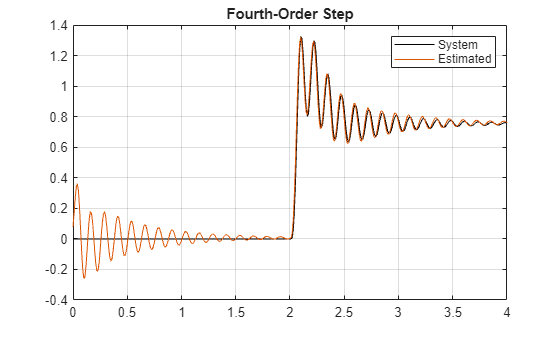### Adjusting fit and initialising network

From the step response of the network in the previous section it is clear that there are still two issues with the fit: first, the network's prediction has a slight offset. In addition to this, the initial state of the network is not stationary which results in transient behaviour at the start of the signal. Initializing the network state to the correct initial condition requires that one update the network state to correspond to the state of the system at the start of the test signal.

While adjusting the initial state of the network, the estimated response of the system at the initial condition is compared to the actual response of the system. The difference between the network's estimation of the initial state and the actual response of the system at the initial state can be used to correct for the offset that is present in the system’s estimations.

#### Setting the network's initial state

When the network is presented with a step input, the initial values of the cell and the hidden state of the network drift towards the correct initial condition. To visualize this, the cell and hidden state of the network is extracted at every time step using the `predictAndUpdateState` function.

Since the step only occurs at 2 seconds, we are interested only in the cell and hidden state values for the first two seconds. A time marker for 2 seconds is defined, and the hidden and cell states are then extracted up to this time marker.

```stepMarker = time <= 2; yhat = zeros(sum(stepMarker),1); hiddenState = zeros(sum(stepMarker),200); %200 LTSM units cellState = zeros(sum(stepMarker),200); for ntime = 1:sum(stepMarker) [fourthOrderNet,yhat(ntime)] = predictAndUpdateState(fourthOrderNet,stepSignal(ntime)'); hiddenState(ntime,:) = fourthOrderNet.Layers(2,1).HiddenState; cellState(ntime,:) = fourthOrderNet.Layers(2,1).CellState; end```

Next plot the hidden and cell states over the period before the step.

```figure subplot(2,1,1) plot(time(1:200),hiddenState(1:200,:)) grid on axis tight title('Hidden State') subplot(2,1,2) plot(time(1:200),cellState(1:200,:)) grid on axis tight title('Cell State')```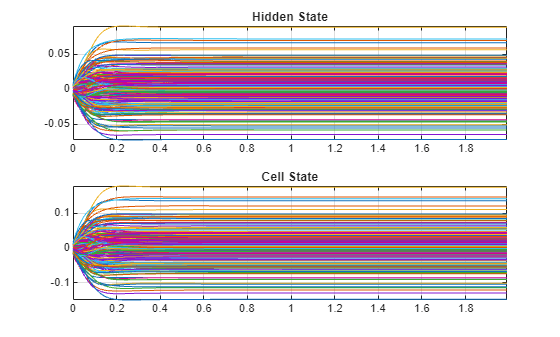To initialize the network for a particular state, an initial output value of zero is chosen. The duration of the signal needs to be long enough for the system to reach a steady state after a disturbance has occurred.

```initializationSignalDuration = 10; %second initializationValue = 0; initilizationSignal = initializationValue*ones(1,initializationSignalDuration*fs); fourthOrderNet = predictAndUpdateState(fourthOrderNet,initilizationSignal);```

Even with the correct initial condition when the network is given a zero signal, the output is not zero. This is because of an incorrect bias term learned by the algorithm during training process.

```figure zeroMapping = predict(fourthOrderNet,initilizationSignal); plot(zeroMapping) axis tight```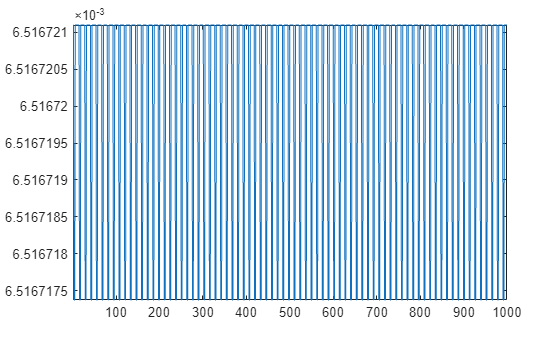Following this adjustment, the network is again tasked to predict the step response. This time the initial disturbance is gone.

```fourthOrderMixedNetStep = predict(fourthOrderNet,stepSignal); figure title('Step response estimation') plot(time,systemResponse,'k', ... time,fourthOrderMixedNetStep,'b') grid on legend('System','Estimated') title('Fourth Order Step - Adjusted State')```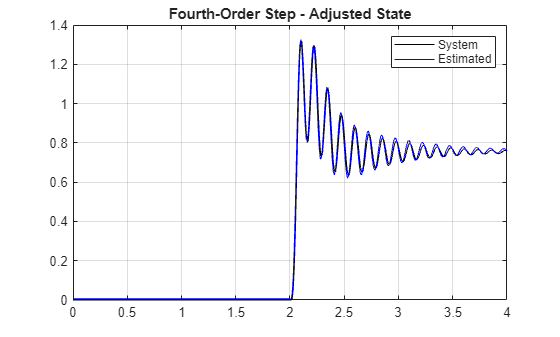Although the network has been adjusted to compensate for the initial condition of the test signal, a small offset is still visible in the predicted response. This is due to the bias term learned by the LSTM during training. This offset is not the reason for the transient state problem addressed in the previous section, since if the network is given a step with the adjusted offset taken into account the transient behaviour is still present. Furthermore, padding the training signal with zeros, centering the training and testing data, or adding a constant zero validation signal does not correct for this. The offset can be fixed by using the same initialization signal that was used for updating the network states. The initialization signal is expected to map the network to zero. The offset between zero and the network estimation is the error in the bias term learned by the network. The offset can be adjusted using this calculated value. Summing the bias term calculated at each layer comes close to the bias detected in the response. Adjusting for the network bias term after the fact however is easier than adjusting the individual bias terms in each layer of the network.

```bias = mean(predict(fourthOrderNet,initilizationSignal)); fourthOrderMixedNetStep = fourthOrderMixedNetStep-bias; figure title('Step response estimation') plot(time,systemResponse,'k',time,fourthOrderMixedNetStep,'b-') legend('System','Estimated') title('Fourth Order Step - Adjusted Offset')```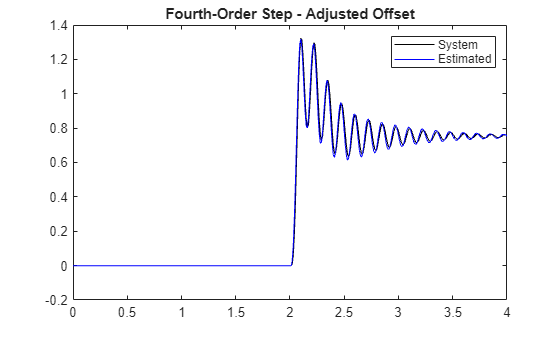### Moving outside of the training range

All the signals used to train the network had a maximum amplitude of 1 and the step function had an amplitude of 0.1. In this section, the behaviour of the network when moving outside of these ranges is investigated.

#### Time Shift

Time shift is introduced by adjusting the time of the step. In the training set it was set to 2 seconds. Here it is set to 3 seconds.

```stepTime = 3; % In seconds stepAmplitue = 0.1; stepDuration = 5; % In seconds [stepSignal,systemResponse,time] = generateStepResponse(fourthOrderMdl,stepTime,stepAmplitue,stepDuration); fourthOrderMixedNetStep = predict(fourthOrderNet,stepSignal); bias = fourthOrderMixedNetStep(1) - initializationValue; fourthOrderMixedNetStep = fourthOrderMixedNetStep-bias; figure plot(time,systemResponse,'k', time,fourthOrderMixedNetStep,'b') grid on axis tight```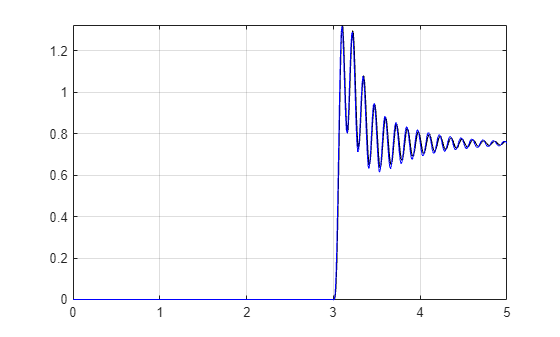#### Amplitude shift

Next the amplitude of the step function is increased to investigate the network's behaviour as the system inputs move outside of the range of the training data. To measure the drift outside of the training data range, the probability density function of the amplitudes in the Gaussian noise signal is measured. The amplitudes are represented as a histogram below:

```figure histogram(urgs,'Normalization','pdf') grid on```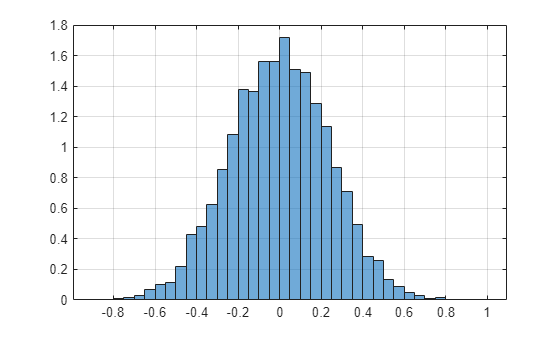Next, the amplitude of the step function is set according to the percentile of the distribution. A plot of error rate as a function of the percentile is also presented.

```pValues = [60:2:98, 90:1:98, 99:0.1:99.9 99.99]; stepAmps = prctile(urgs,pValues); % Amplitudes stepTime = 3; % In seconds stepDuration = 5; % In seconds stepMSE = zeros(length(stepAmps),1); fourthOrderMixedNetStep = cell(length(stepAmps),1); steps = cell(length(stepAmps),1); for nAmps = 1:length(stepAmps) % Fourth order mixed [stepSignal,systemResponse,time] = generateStepResponse(fourthOrderMdl,stepTime,stepAmps(nAmps),stepDuration); fourthOrderMixedNetStep{nAmps} = predict(fourthOrderNet,stepSignal); bias = fourthOrderMixedNetStep{nAmps}(1) - initializationValue; fourthOrderMixedNetStep{nAmps} = fourthOrderMixedNetStep{nAmps}-bias; stepMSE(nAmps) = sqrt(sum((systemResponse-fourthOrderMixedNetStep{nAmps}).^2)); steps{nAmps,1} = systemResponse; end figure plot(pValues,stepMSE,'bo') title('Prediction Error as a function of deviation from training range') grid on axis tight``````subplot(2,1,1) plot(time,steps{1},'k', time,fourthOrderMixedNetStep{1},'b') grid on axis tight title('Best Performance') xlabel('time') ylabel('System Response') subplot(2,1,2) plot(time,steps{end},'k', time,fourthOrderMixedNetStep{end},'b') grid on axis tight title('Worst Performance') xlabel('time') ylabel('System Response')```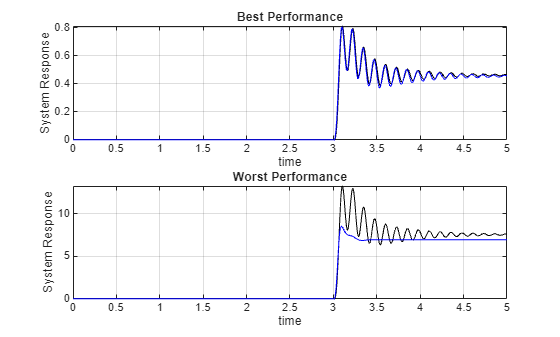As the amplitude of the step response moves outside of the range of the training set, the LSTM attempts to estimate the average value of the response.

### Changing System bandwidth

In this section we look briefly of the effect the system bandwidth on the number of hidden units select for the LSTM. This is done by modelling the fourth-order mixed-dynamics transfer function with four different networks:

• a small network which has 5 hidden units and a single LSTM layer,

• a medium network with 10 hidden units and a single LSTM layer,

• a full network with 100 hidden units and a single LSTM layer,

• a deep network with two LSTM layers (each with 100 hidden units).

These networks were trained and had their states updated in the same way as described above. Load the trained networks

`load('variousHiddenUnitNets.mat')`

Generate step signal

```stepTime = 2; % In seconds stepAmplitue = 0.1; stepDuration = 4; % In seconds % Constuct step signal time = (0:1/fs:stepDuration)'; stepSignal = [zeros(sum(time<=stepTime),1);stepAmplitue*ones(sum(time>stepTime),1)]; systemResponse = lsim(fourthOrderMdl,stepSignal,time); % Transpose input and output signal for network inputs. stepSignal = stepSignal'; systemResponse = systemResponse';```

Estimate the system response using the various trained networks

```smallNetStep = predict(smallNet,stepSignal)-smallNetZeroMapping(end); medNetStep = predict(medNet,stepSignal)-medNetZeroMapping(end); fullnetStep = predict(fullNet,stepSignal) - fullNetZeroMapping(end); doubleNetStep = predict(doubleNet,stepSignal) - doubleNetZeroMapping(end); ```

Plot the estimate response

```figure title('Step response estimation') plot(time,systemResponse,'k', ... time,doubleNetStep,'g', ... time,fullnetStep,'r', ... time,medNetStep,'c', ... time,smallNetStep,'b') grid on legend({'System','Double Net','Full Net','Med Net','Small Net'},'Location','northwest') title('Fourth Order Step')```A moving average of the responses are plotted in order to compare the slow varying dynamics of the system. The ability of the LSTM to capture the long-term dynamics of the linear system is directly related to the dynamics of the system and the number iof hidden units in the LSTM. The number of layers in the LSTM is not directly related to the long-term behaviour but rather adds flexibility to adjust the estimation from the first layer.

```figure title('Slow dynamics component') plot(time,movmean(systemResponse,50),'k') hold on plot(time,movmean(doubleNetStep,50),'g') plot(time,movmean(fullnetStep,50),'r') plot(time,movmean(medNetStep,50),'c') plot(time,movmean(smallNetStep,50),'b') grid on legend('System','Double Net','Full net','Med Net','Small Net','Location','northwest') title('Fourth Order Step')```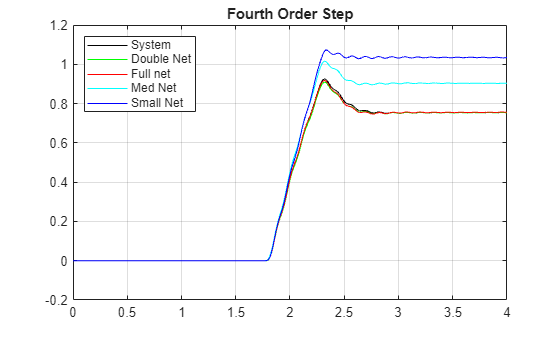In this section random noise is added to the output of the system response. The purpose is to explore the effect of noise on the LSTM performance. To this end, white noise with levels of 1%, 5% and 10% was added to the measured system responses. To assess the robustness of LSTMs to noise the `tfest` function is used as a baseline to compare the estimations against. The LSTM and `tfest` is applied to the same datasets.

The step function from before is used:

```stepTime = 2; % In seconds stepAmplitue = 0.1; stepDuration = 4; % In seconds [stepSignal,systemResponse,time] = generateStepResponse(fourthOrderMdl,stepTime,stepAmplitue,stepDuration);```

Load trained networks and estimate the system's response

```load('noisyDataNetworks.mat') netNoise1Step = predictAndAdjust(netNoise1,stepSignal,initilizationSignal,initializationValue); netNoise5Step = predictAndAdjust(netNoise5,stepSignal,initilizationSignal,initializationValue); netNoise10Step = predictAndAdjust(netNoise10,stepSignal,initilizationSignal,initializationValue);```

A transfer function estimator (`tfest`) is used to estimate the function at the above noise levels to compare the resilience of the networks to noise (see the accompanying `noiseLevelModels.m` for more details).

```load('noisyDataTFs.mat') tfStepNoise1 = lsim(tfNoise1,stepSignal,time); tfStepNoise5 = lsim(tfNoise5,stepSignal,time); tfStepNoise10 = lsim(tfNoise10,stepSignal,time); ```

Plot the generated responses.

```figure plot(time,systemResponse,'k', ... time,netNoise1Step, ... time,netNoise5Step, ... time,netNoise10Step) grid on legend('System Response','1% Noise','5% Noise','10% Noise') title('Deep LSTM with noisy data')```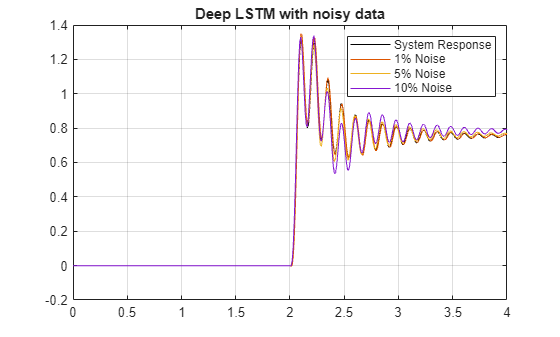A similar plot is made for the fitted transfer functions.

```figure plot(time,systemResponse,'k', ... time,tfStepNoise1, ... time,tfStepNoise5, ... time,tfStepNoise10) grid on legend('System Response','1% Noise','5% Noise','10% Noise') title('Transfer functions fitted to noisy data')```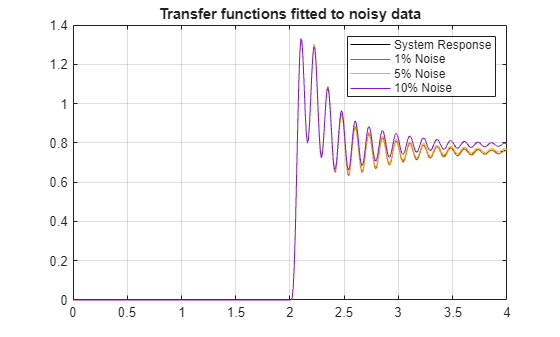The MSE (mean square error) is also calculated to better assess the performance of the different models for different noise levels.

```msefun = @(y,yhat) mean(sqrt((y-yhat).^2)/length(y)); % LSTM errors lstmMSE(1,:) = msefun(systemResponse,netNoise1Step); lstmMSE(2,:) = msefun(systemResponse,netNoise5Step); lstmMSE(3,:) = msefun(systemResponse,netNoise10Step); % Transfer function errors tfMSE(1,:) = msefun(systemResponse,tfStepNoise1'); tfMSE(2,:) = msefun(systemResponse,tfStepNoise5'); tfMSE(3,:) = msefun(systemResponse,tfStepNoise10'); mseTbl = array2table([lstmMSE tfMSE],'VariableNames',{'LSTMMSE','TFMSE'})```
```mseTbl=3×2 table LSTMMSE TFMSE __________ __________ 1.0115e-05 8.8621e-07 2.5577e-05 9.9064e-06 5.1791e-05 3.6831e-05 ```

### References

 1] Gers, Felix A., Jürgen Schmidhuber, and Fred Cummins. “Learning to Forget: Continual Prediction with LSTM.” Neural Computation 12, no. 10 (October 2000): 2451–71.

 Graves, Alex, Santiago Fernández, and Jürgen Schmidhuber. “Bidirectional LSTM Networks for Improved Phoneme Classification and Recognition.” International Conference on Artificial Neural Networks (September 2005): 799–804. Warsaw, Poland: Springer.

 Graves, Alex, Abdel-rahman Mohamed, and Geoffrey Hinton. “Speech Recognition with Deep Recurrent Neural Networks.” IEEE International Conference on Acoustics, Speech, and Signal Processing (March, 2013):6645–6649. IEEE.

 Hochreiter, Sepp. “The Vanishing Gradient Problem During Learning Recurrent Neural Nets and Problem Solutions.” International Journal of Uncertainty, Fuzziness and Knowledge-Based Systems 06, no. 02 (April 1998): 107–16.

### Helper functions

```function [stepSignal,systemResponse,time] = generateStepResponse(model,stepTime,stepAmp,signalDuration) %Generates a step response for the given model. % %Check model type modelType = class(model); if nargin < 2 stepTime = 1; end if nargin < 3 stepAmp = 1; end if nargin < 4 signalDuration = 10; end % Constuct step signal if model.Ts == 0 Ts = 1e-2; time = (0:Ts:signalDuration)'; else time = (0:model.Ts:signalDuration)'; end stepSignal = [zeros(sum(time<=stepTime),1);stepAmp*ones(sum(time>stepTime),1)]; switch modelType case {'tf', 'zpk'} systemResponse = lsim(model,stepSignal,time); case 'idpoly' systemResponse = sim(model,stepSignal,time); otherwise error('Model passed is not supported') end stepSignal = stepSignal'; systemResponse = systemResponse'; end```
##### SupportGet trial now